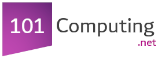Start

End
Create a program that will calculate the following sum:
1 + 2 + 3 + 4 + ... + 98 + 99 + 100 = ?
total = 0
for i in range(1,101):
total = total + i
print("1+2+3+...+99+100 = ")
print(total)